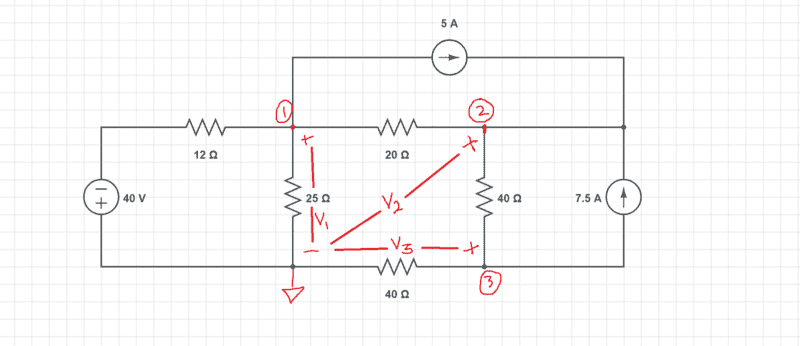# Why do you subtract different voltages in NodeVoltageMethod

AchillesWrathfulLove
Derived from this circuits 3 nodes are 3 equations using the NodeVoltageMethod

1. (V1--40)/12 + 5 + (V1-V2)/20 + V1/25 = 0
2. (V2-V1)/20 + (V2-V3)/40 - 7.5 - 5 = 0
3. V3/40 + (V3-V2)/40 + 7.5 = 0

The only pattern I can see here is that if it is at node 1 then it is always the node voltage belonging to node 1 (which is V1) minus something else. I know that "this" minus "this" gives you the voltage across the horizontal or sometimes vertical resistor but I don't know WHY. Why do you minus voltages from each other.#### Attachments

Mentor
What does you textbook says about the method? What sources have you tried to consult to answer the question and to learn how to apply the method?

Looks to me like you are searching for a pattern in worked out examples and reinventing the wheel instead of just learning the basics of the method, where it is all explained.

Gold Member
Dearly Missed
Did you derive those three equations or is that from an example problem out of your course material ?

Why do you minus voltages from each other.
Short answer is because voltage is a DIFFERENCE (remember definition of voltage, it's Potential Difference) and to get a difference you subtract. You're finding the voltage across those 12, 20 , and 40 ohm resistors.

I know that sounds like a wise-guy answer but it's not.

I don't want to start in on my long answer because i was taught to treat current entering a node as positive, current leaving as negative,
yet i notice your 5 and 7.5 amp current sources are assigned a + sign when leaving nodes 1 and 3 and a - sin when entering node 2.

So i'll wait and see if anybody clarifies that conundrum..
Might be as simple as in 1961 when i was taught, we still believed in "electron current" and our textbook was the RCA Receiving Tube Manual.

That's why i asked if those equations were yours or a textbook author's.
Each author is free to set his conventions provided he clearly defines them.
I don't want to mix different sets of conventions--- that's chaos.

old jim

AchillesWrathfulLove
I don't want to start in on my long answer because i was taught to treat current entering a node as positive, current leaving as negative,

Well i was taught that current into node is negative and current out of node is positive so that is why my equations are as such. Thank you for your answer.